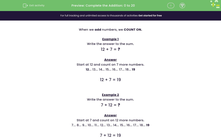# Complete the Addition: 0 to 20

In this worksheet, students will practise their addition skills.Key stage:  KS 1

Curriculum topic:   Number: Addition and Subtraction

Curriculum subtopic:   Add and Subtract to 20

Difficulty level:#### Worksheet Overview

When we add numbers, we count on.

Example 1

Write the answer to the sum:

12 + 7 = ?

Start at 12 and count on 7 more numbers.

The first one is 13, then 14 and so on, 15... 16... 17... 18... 19

Remember not to count the number you started from, which was 12 in this question.

12 + 7 = 19

Example 2

Write the answer to the sum.

7 + 12 = ?

Start at 7 and count on 12 more numbers.

The first number will be 8, then 9 and so on, 10... 11... 12... 13... 14... 15... 16... 17... 18... 19

Again, don't count the number you started from - in this case 7.

7 + 12 = 19

Did you spot that 12 + 7 and 7 + 12 give the same answer?

If you know the answer to one of those sums, you have been given the answer to the other one for no extra work!

That has to be good!Are you ready to have a go at some questions?

### What is EdPlace?

We're your National Curriculum aligned online education content provider helping each child succeed in English, maths and science from year 1 to GCSE. With an EdPlace account you’ll be able to track and measure progress, helping each child achieve their best. We build confidence and attainment by personalising each child’s learning at a level that suits them.

Get started••••